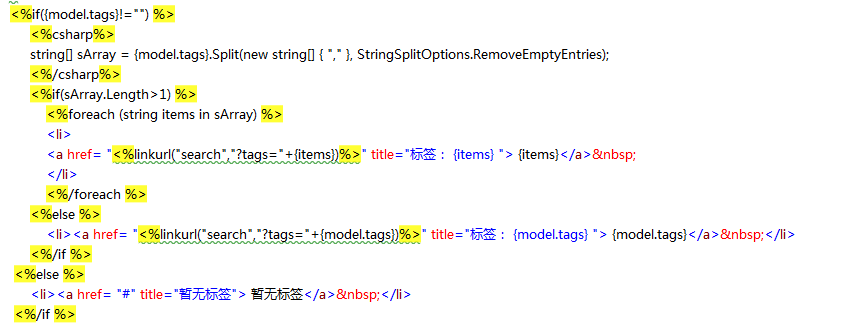### 1.利用javascript脚本语言，通过for循环输出：

```<script type="text/javascript">
var col = "{dr[tags]}";
var arr = col.split(",");
for (i = 0; i < arr.length; i++) {
if (col != "") {
document.write("<a href='<%linkurl("search")%>?tags=" + arr[i] + "'>" + arr[i] + "</a>");
};
}
</script>```

### 2.在html页面中写csharp方法：

```<%csharp%>
string str1 = {dr[tags]};
string[] arr1 = str1.Split(',');
string tags1 = "";
for (int i = 0; i < arr1.Length; i++){
tags1 +="<a href='" + linkurl("search","?tags="+arr1[i].ToString()) + "'>"+arr1[i]+"</a>";
}
<%/csharp%>
{tags1}```